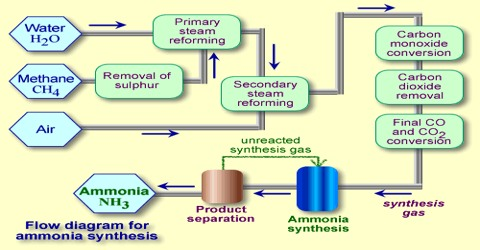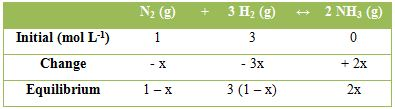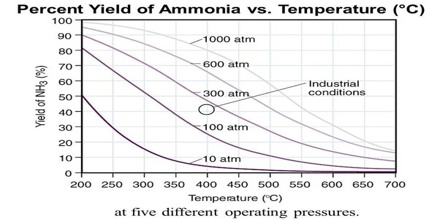Chemistry

# Applications of Principles of Chemical Equilibrium: Synthesis of AmmoniaApplications of the Principles of Chemical Equilibrium to Reactions of Industrial Importance

In industrial processes the primary objective is to maximize the yield of products at a minimum cost in a shorter period of time. To attain this objective in the case of gaseous reactions of industrial importance advantage is taken of the influence of pressure and temperature on the equilibrium yield of the desired product. These points will be illustrated with the help of a few industrially important reactions.

Synthesis of ammonia

The synthesis of ammonia on a commercial scale by the Haber-Bosch process is based on following reaction:

N2 (g) + 3 H2 (g) ↔ 2 NH3 (g)

[∆H291 = – 92.0 kJ and ∆H294 = – 111.2 kJ]

For, the calculation of the equilibrium constant, let the concentration (mol L-1) of the various species at equilibrium be as follow:hence, KC = 2x2 / [(1 – x) (3 – 3x)3]

Total number of moles at equilibrium = 1 – x + 3(1 – x) + 2x = 4 – 2x

Where, x is the number of moles of N2 reacted. Let the equilibrium process be P atmosphere. The mole fractions of the components are,

xN2 = (1 – x) / (4 – 2x); xH2 = (3 – 3x) / (4 – 2x) and NH3 = 2x / (4 – 2x)

The reaction is a highly exothermic one and as equation (10.28) shows the equilibrium yield of ammonia depends on the pressure to a large extent. The suitable reaction conditions are low temperatures and high pressures. Some of the experimental results for this traction are shown in Figure.Fig: Yield of ammonia at different temperatures and pressures

At low temperature, however, the reaction is slow and the time to attain the desired yield is long. For the process to be economical the reaction – should be conducted at the lowest temperature at which the reaction would proceed at the desired speed. In such industrial processes advantage is taken of the catalytic effect of certain substances in increasing the speed of reaction. Iron with the addition of small amounts of the oxides of aluminum and potassium acts as good catalyst. For all catalytic reactions there is an optimum temperature at which the rate is such that the yield of the product is best for the cost involved.

As the reaction involves a decrease of volume, the yield of ammonia increases with increase of pressure. In the actual manufacturing process by the Haber-Bosch process a pressure of about 200 atm and temperature of about 450° to 550°C’ are used. In other modifications of the process, pressure as high as 1000 atmosphere is used.